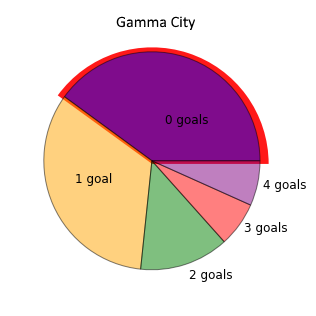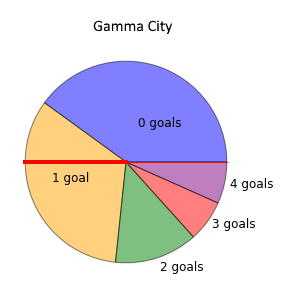#### You may also like### Jewellery Boxes

Can you find the value of the jewels?### Average Discovery

Find the missing number if the mean, median and mode are all the same.### Algebraic Average

The mean of three numbers x, y and z is x. What is the mean of y and z?

# Gamma City

##### Age 14 to 16 Short Challenge Level:

The mode is the most common number of goals scored per match.
On the pie chart, this will be the 'fattest' wedge:Mode number of goals = 0

The median is the middle value, when the data is in order. The pie charts begin from 0 goals, and the number of goals increases in an anticlockwise direction, so the pie chart shows the data in order.

After 180$^\circ$, the middle value is reached, so the wedge that the 180$^\circ$ line lands in is the median.Median number of goals = 1

You can find more short problems, arranged by curriculum topic, in our short problems collection.